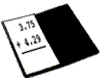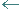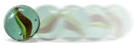# Estimating Decimal SumsExample 1: Charlene and Margi went to a nearby restaurant for a quick lunch. The waitress handed them the bill with the price of each lunch, but forgot to add up the total. Estimate the total bill if Charlene's lunch was \$3.75 and Margi's lunch was \$4.29.

Analysis: Estimation is a good tool for making a rough calculation. There are many estimation strategies that you could use to estimate the sum of these decimals. Let's look at the front-end strategy and the rounding strategy. We will round to the nearest tenth.

Estimates:

Front-End Strategy

3.75      3.75about
4.29   + 4.291
7         7 + 1 = 8

3 + 4 = 7 and .75 plus .29 is about 1. Thus, \$7+ \$1 = \$8. The estimated sum is \$8.

Rounding Strategy

Round each decimal to a designated place value, then add to estimate the sum.

3.73.8
+ 4.2+ 4.3
+ 4.29+    8.1

Rounding each decimal to the nearest tenth, we get an estimated sum of 8.1 or \$8.10.

Answer: Both \$8 and \$8.10 are good estimates for the total lunch bill for Charlene and Margi.

Note the difference between the two strategies used in Example 1: The front-end strategy uses the first digit to estimate a sum and then considers the other digits to adjust the estimate. In the rounding strategy, addition does not occur until after the numbers have been rounded. In both strategies, you must line up both decimals before proceeding.

Procedure: To round a decimal to a designated place value, first underline or mark that place. If the digit to the right of that place is 5 through 9, then round up. If the digit to the right of that place is 1 through 4, then round down, leave the digit in the designated place unchanged, and drop all digits to the right of it.

Let's look at some more examples of estimating decimal sums.

Example 2: Estimate the sum of each pair of decimals by rounding to the specified place.

A. Estimate 4.203 + 6.598 by rounding to the nearest hundredth.

4.204.20
+ 6.598+ 6.60
10.80

 If the digit to the right of the place you are rounding to is 1 through 4, then round down. Thus, 4.203 is rounded down to 4.20. If the digit to the right of the place you are rounding to is 5 through 9, then round up. Thus, 6.598 is rounded up to 6.60.

B. Estimate \$12.96 + \$7.19 by rounding to the nearest one.

\$12.96\$13
+ \$  7.19+ \$ 7
\$20

 If the digit to the right of the place you are rounding to is 5 through 9, then round up. Thus, 12.96 is rounded up to 13. If the digit to the right of the place you are rounding to is 1 through 4, then round down. Thus, 7.19 is rounded down to 7.

C. Estimate 11.79 + 4.58 by rounding to the nearest tenth.

11.711.8
+   4.58+  4 6
16.4

 If the digit to the right of the place you are rounding to is 5 through 9, then round up. Thus, 11.79 is rounded up to 11.8. If the digit to the right of the place you are rounding to is 5 through 9, then round up. Thus, 4.58 is rounded up to 4.6.

Example 3: Estimate the sum of each set of decimals by using the front-end strategy.

A: Estimate 1.93 + 5.248

1.93        1.930about
5.248   + 5.2481.2
6             6 + 1.2 = 7.2

1 + 5 = 6 and .930 + .248 is about 1.2. Thus, 6 + 1.2 = 7.2 The estimated sum is 7.2

B. Estimate 4.23 + 4.009 + 4.54 + 3.89

4.230       4.230about
4.009       4.009                                 .

4.540       4.540                                 .
+  3.890   +  3.8901.5
15        15 + 1.5 = 16.5

4 + 4 + 4 + 3 = 15 and .230 + .009 + .540 + .890 is about 1.5. Thus, 15 + 1.5 = 16.5. The estimated sum is 16.5.

Example 4: Use either method to estimate the sum of  6.37 + 2.8 + 21.19

Front-End Strategy

6.37              6.37about
2.80              2.80                        .
+   21.19        + 21.191
29               29 + 1 = 30

Answer: 6 + 2 + 21 = 29 and.37 + .80 + .19 is about 1. Thus, 29 + 1 = 30. The estimated sum is 30.

Rounding Strategy

6.36.4
2.82.8
+21.1+  21.2
30.4

Rounding each decimal to the nearest tenth, we get an estimated sum of 30.4.

As you can see from our examples, estimates will vary depending on which strategy is used. In Example 4, we got an estimated sum of 30 using the front-end strategy, and an estimate of 30.4 by rounding to the nearest tenth. The actual sum is 30.36, thus 30 is an underestimate and 30.4 is an overestimate.

Definition 1: An overestimate is an an estimate that is too high: it exceeds the actual answer.

Definition 2: An underestimate is an estimate that is too low: it is lower than the actual answer.

Example 5: Estimate the sum of each pair of decimals by rounding to the nearest tenth. Then indicate if the estimate is an overestimate or an underestimate by comparing it to the actual answer.

Estimate

A.

\$36.23+   \$36.20
+ \$63.44+ \$63.40
+ \$99.60+      \$99.60

Actual Sum = \$99.67

Overestimate or Underestimate? Underestimate since \$99.60 < \$99.67

B.

\$57.6\$ 57.70
+ \$43.31+  \$ 43.30\$101.00

Actual Sum = \$100.96

Overestimate or Underestimate? Overestimate since \$101.00 > \$100.96

C.

\$24.8\$24.90
+ \$72.15+ \$72.20\$97.10

Actual Sum = \$97.04

Overestimate or Underestimate? Overestimate since \$97.10 > \$97.04Example 6: A marble rolled 3.9658 cm and then rolled 16.37 cm. Jen estimated that the marble rolled 20 cm altogether. If the actual sum is 20.3358 cm, did she overestimate or underestimate? Explain your answer.

Answer: Jen underestimated because her estimate of 20 cm was lower than the actual sum of 20.3358 cm.

Summary: Estimation is a good tool for making a rough calculation. In this lesson, we learned how to estimate decimal sums using two different strategies: front-end and rounding. In both strategies, you must line up all decimals before proceeding. An overestimate is an an estimate that is too high; an underestimate is an estimate that is too low.

### Exercises

In Exercises 1 through 3, you may use paper and pencil to help you estimate. Click once in an ANSWER BOX and type in your answer; then click ENTER. After you click ENTER, a message will appear in the RESULTS BOX to indicate whether your answer is correct or incorrect. To start over, click CLEAR.

 1.Estimate the sum of 0.79 and 0.13 by rounding to the nearest tenth. ANSWER BOX:   RESULTS BOX:
 2.Estimate the sum of 3.197 and 4.214 by rounding to the nearest hundredth. ANSWER BOX:   RESULTS BOX:
 3.Estimate the sum of 1.768 and 2.209 using the front-end strategy. ANSWER BOX:   RESULTS BOX:
 In Exercises 4 and 5, read each question below. Select your answer by clicking on its button. Feedback to your answer is provided in the RESULTS BOX. If you make a mistake, choose a different button.
 4.The sum of two decimals is 56.9531. If Aaron overestimated the sum, then which of the following estimates did he use? 56.95356.9556.957 RESULTS BOX:
 5.The sum of two decimals is 31.5487. If Lana underestimated the sum, then which of the following estimates did she use? 31.531.5531.54932 RESULTS BOX: